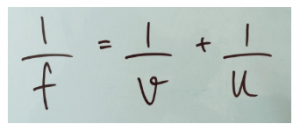Checkout JEE MAINS 2022 Question Paper Analysis : Checkout JEE MAINS 2022 Question Paper Analysis :

# Thin Lens Formula for Concave and Convex Lenses

## Thin Lens Formula with Magnification for Concave and Convex Lenses

First, what is a thin lens? If the thickness of a lens is negligible in comparison to the radius of curvature, it is a thin lens. The following typical equation is used to determine a relation between the focal length of the lens, the distance of the object, and the distance of the image.Where,

$$\begin{array}{l}f\end{array}$$
is the focal length

$$\begin{array}{l}v\end{array}$$
is the distance of the image from the optical center

$$\begin{array}{l}u\end{array}$$
is the distance of the object from the optical center

If the focal length is negative, it implies that the lens is concave (diverging), while if positive it implies that the lens is convex (converging lenses). If the image distance is calculated to be negative, it implies that the image formed is virtual and on the same side as the object.

The following video explains the thin lens formula:The magnification (

$$\begin{array}{l}M\end{array}$$
) of the image formed can be calculated using the following formula.Where,

$$\begin{array}{l}v\end{array}$$
is the object height

$$\begin{array}{l}u\end{array}$$
is the image height

If ‘

$$\begin{array}{l}i\end{array}$$
’ is positive, the image is upright and if ‘
$$\begin{array}{l}i\end{array}$$
’ is negative, the image is inverted.

The two formulas given above are together referred to as the thin lens formula. You can try the following sample problem using this.

A 4 cm tall candle is placed in front of a double convex lens. The lens has a focal length of 15.2 cm. The object has been placed 45.7 cm from the vertex. Where will the image be formed and what will its size be?

Ans: Image distance = 22.8 cm; Image Height = -1.99 cm

Solve application questions using a thin lens formula by joining other students here at BYJU’S.

## Frequently Asked Questions – FAQs

### What is a lens?

A lens is a piece of transparent material or glass with curved sides for dispersing or focusing light rays.

### What are the two common types of lenses?

The two most common types of lenses are convex and concave lenses.

### What is a contact lens?

A contact lens is a specially designed thin lens placed directly above the viewing plane of the eyes. They are worn to correct vision problems or for therapeutic and cosmetic reasons.

### What is the connection between the focal length and the refractive index of a lens?

The focal length of a lens depends on the refractive index of the lens and the radii of curvature.

### What are the important uses of lenses?

Lenses are employed in different imaging tools like cameras, binoculars, and telescopes. They are also extensively used for correcting vision defects such as hypermetropia and myopia.

Test Your Knowledge On Thin Lens Formula For Concave And Convex Lenses!